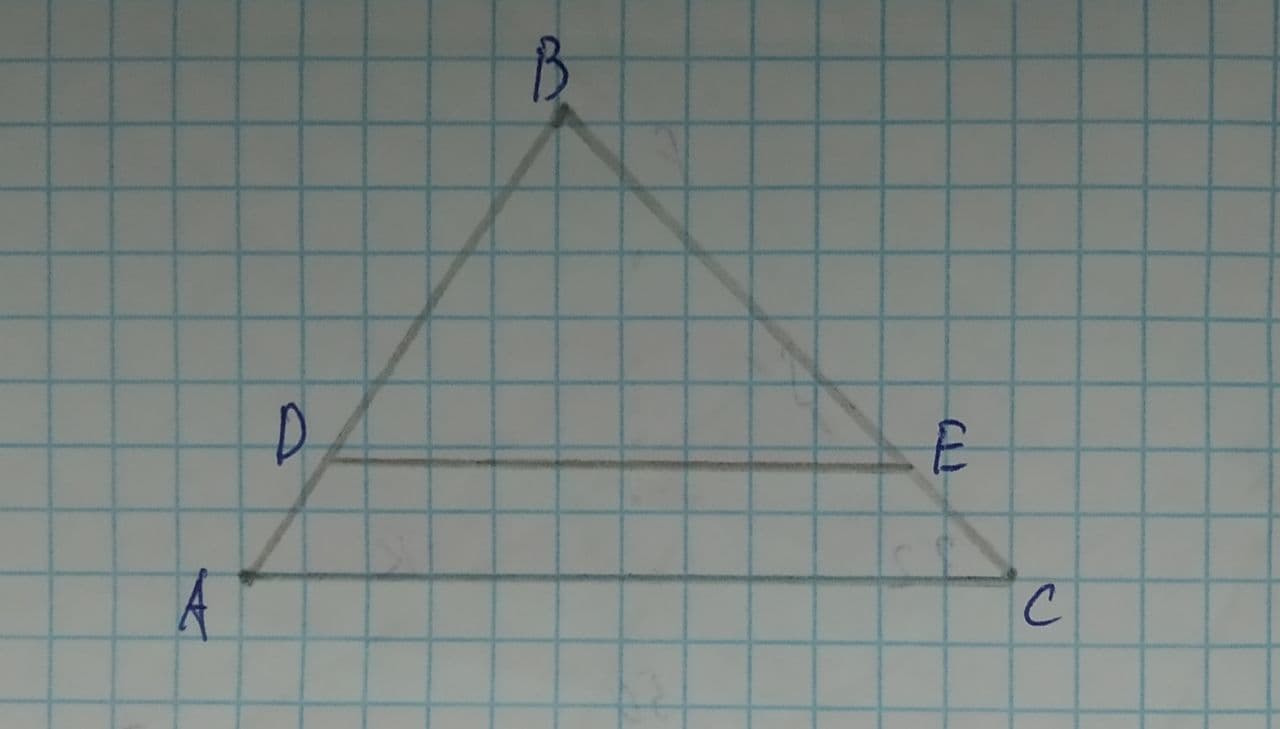# Consider the following figure where \triangle ABC \sim \triangle DBE AC=16, CB=10abondantQ 2021-08-11 Answered
Consider the following figure where $$\displaystyle\triangle{A}{B}{C}\sim\triangle{D}{B}{E}$$.Given: AC=16, CB=10
E is the midpoint of $$\displaystyle\overline{{{C}{B}}}$$
Find: DE

• Questions are typically answered in as fast as 30 minutes

### Plainmath recommends

• Get a detailed answer even on the hardest topics.
• Ask an expert for a step-by-step guidance to learn to do it yourself.crocolylec
Step 1
Explanation:
Given that,
$$\displaystyle\triangle{A}{B}{C}\sim\triangle{D}{B}{E}$$
AC=16
CB=10
E is the midpoint of CB
E is the midpoint, it means
BE=EC=5
We need to find the DE
Step 2
According to the triangle's similarity, the corresponding ratio of sides is the same.
$$\displaystyle{\frac{{{D}{E}}}{{{A}{C}}}}={\frac{{{B}{E}}}{{{C}{B}}}}$$
Suppose DE=x
So,
$$\displaystyle{\frac{{{x}}}{{{16}}}}={\frac{{{5}}}{{{10}}}}$$
$$\displaystyle{x}={\frac{{{5}}}{{{10}}}}\times{16}$$
x=8
Hence, the DE=8